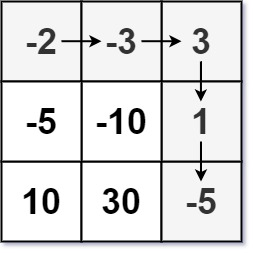## Algorithm

Problem Name: 174. Dungeon Game

The demons had captured the princess and imprisoned her in the bottom-right corner of a `dungeon`. The `dungeon` consists of `m x n` rooms laid out in a 2D grid. Our valiant knight was initially positioned in the top-left room and must fight his way through `dungeon` to rescue the princess.

The knight has an initial health point represented by a positive integer. If at any point his health point drops to `0` or below, he dies immediately.

Some of the rooms are guarded by demons (represented by negative integers), so the knight loses health upon entering these rooms; other rooms are either empty (represented as 0) or contain magic orbs that increase the knight's health (represented by positive integers).

To reach the princess as quickly as possible, the knight decides to move only rightward or downward in each step.

Return the knight's minimum initial health so that he can rescue the princess.

Note that any room can contain threats or power-ups, even the first room the knight enters and the bottom-right room where the princess is imprisoned.

Example 1:```Input: dungeon = [[-2,-3,3],[-5,-10,1],[10,30,-5]]
Output: 7
Explanation: The initial health of the knight must be at least 7 if he follows the optimal path: RIGHT-> RIGHT -> DOWN -> DOWN.
```

Example 2:

```Input: dungeon = []
Output: 1
```

Constraints:

• `m == dungeon.length`
• `n == dungeon[i].length`
• `1 <= m, n <= 200`
• `-1000 <= dungeon[i][j] <= 1000`

## Code Examples

### #1 Code Example with Javascript Programming

```Code - Javascript Programming```

``````
const calculateMinimumHP = function (dungeon) {
const M = dungeon.length
const N = dungeon.length
// hp[i][j] represents the min hp needed at position (i, j)
// Add dummy row and column at bottom and right side
const hp = Array.from({ length: M + 1 }, () =>
Array(N + 1).fill(Number.MAX_VALUE)
)
hp[M][N - 1] = 1
hp[M - 1][N] = 1
for (let i = M - 1; i >= 0; i--) {
for (let j = N - 1; j >= 0; j--) {
const need = Math.min(hp[i + 1][j], hp[i][j + 1]) - dungeon[i][j]
hp[i][j] = need <= 0 ? 1 : need
}
}
return hp
}
``````
Copy The Code &

Input

cmd
dungeon = [[-2,-3,3],[-5,-10,1],[10,30,-5]]

Output

cmd
7

### #2 Code Example with Python Programming

```Code - Python Programming```

``````
class Solution:
def calculateMinimumHP(self, dungeon):
m, n = len(dungeon), len(dungeon)
for i in range(m - 1, -1, -1):
for j in range(n - 1, -1, -1):
if i == m - 1 and j == n - 1:
dungeon[i][j] = max(1, 1 - dungeon[i][j])
elif j == n - 1:
dungeon[i][j] = max(1, dungeon[i + 1][j] - dungeon[i][j])
elif i == m - 1:
dungeon[i][j] = max(1, dungeon[i][j + 1] - dungeon[i][j])
else:
dungeon[i][j] = max(1, min(dungeon[i + 1][j], dungeon[i][j + 1]) - dungeon[i][j])
return dungeon
``````
Copy The Code &

Input

cmd
dungeon = []

Output

cmd
1

### #3 Code Example with C# Programming

```Code - C# Programming```

``````
using System;

namespace LeetCode
{
public class _0174_DungeonGame
{
public int CalculateMinimumHP(int[][] dungeon)
{
var rows = dungeon.Length;
var cols = dungeon.Length;

for (int i = rows - 1; i >= 0; i--)
for (int j = cols - 1; j >= 0; j--)
{
if (i == rows - 1 && j == cols - 1) continue;

int rightValue = int.MinValue, downValue = int.MinValue;
var current = dungeon[i][j];

if (i < cols - 1) rightValue = current > 0 ? current + dungeon[i + 1][j] : Math.Min(current, current + dungeon[i + 1][j]);
if (j < rows - 1) downValue = current > 0 ? current + dungeon[i][j + 1] : Math.Min(current, current + dungeon[i][j + 1]);
dungeon[i][j] = Math.Max(rightValue, downValue);
}

return dungeon <= 0 ? 1 - dungeon : 1;
}
}
}
``````
Copy The Code &

Input

cmd
dungeon = []

Output

cmd
1